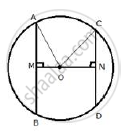Share

Prove That, of Any Two Chords of a Circle, the Greater Chord is Nearer to the Centre. - ICSE Class 10 - Mathematics

ConceptArc and Chord Properties - If Two Chords Intersect Internally Or Externally Then the Product of the Lengths of the Segments Are Equal

Question

Prove that, of any two chords of a circle, the greater chord is nearer to the centre.

SolutionGiven: A circle with centre O and radius r.OM ⊥  AB and ON ⊥ CD Also AB > CD
To prove: OM < ON
Proof: Join OA and OC.
In Rt.  ΔAOM,
AO^2  = AM^2  + OM^2
r^2 = (1/2 AB)^2  + OM^2
r^2 = 1/4 AB^2 + OM^2              ………..(i)
Again in Rt.  ΔONC,
OC^2 = NC^2  + ON^2
⇒ r^2 =  (1/2 CD )^2 + ON^2

 ⇒ r^2 = 1/4  CD^2 + ON^2          ………..(ii)
From (i) and (ii)
1/4 AB^2 + OM^2 = 1/4  CD^2 + ON^2
But, AB > CD (given)
∴ ON > OM
⇒ OM < ON
Hence, AB is nearer to the centre than CD.

Is there an error in this question or solution?

APPEARS IN

Solution Prove That, of Any Two Chords of a Circle, the Greater Chord is Nearer to the Centre. Concept: Arc and Chord Properties - If Two Chords Intersect Internally Or Externally Then the Product of the Lengths of the Segments Are Equal.
S